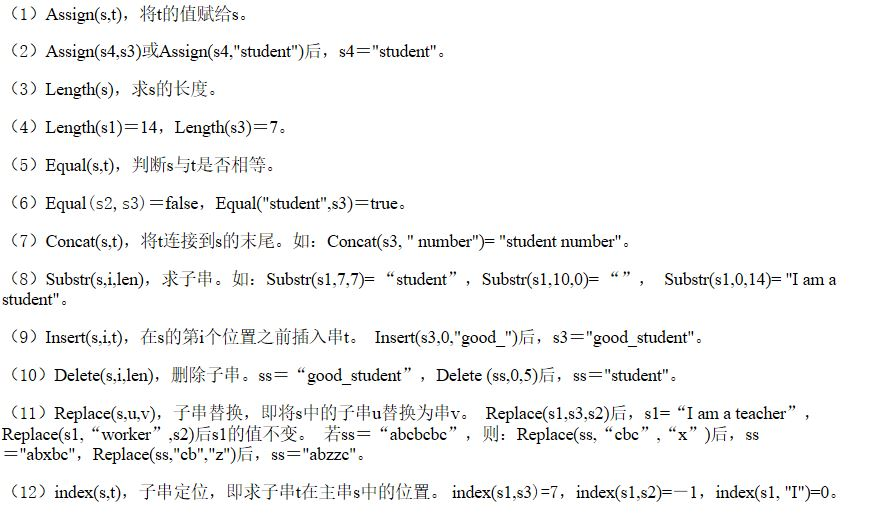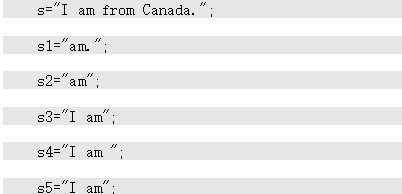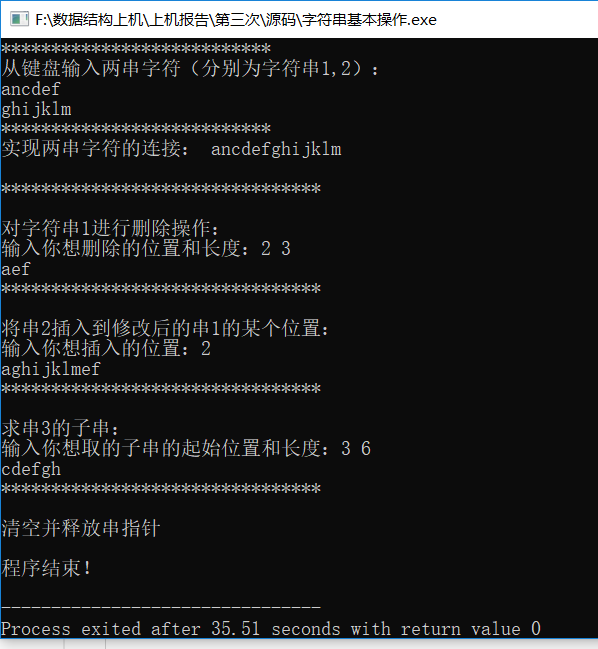• ## 字符串

千次阅读 多人点赞 2020-05-18 09:20:10
字符串的定义：串是字符串的简称。在数据结构中，串是一种在数据元素的组成上具有一定约束...零个字符的串（即：””）称为空串，空串不包含任何字符。值得注意的是： （1）长度为1的空格串" "不等同于空串""； （2）
字符串的定义：串是字符串的简称。在数据结构中，串是一种在数据元素的组成上具有一定约束条件的线性表，即要求组成线性表的所有数据元素都是字符，所以说串是一个有穷的字符序列。

串是由零个或多个字符组成的有限序列，记作s=”s0s1…sn-1”(n≥0)，其中s是串名，字符个数n称作串的长度，双撇号括起来的字符序列”s0s1…sn-1”是串的值。每个字符可以是字母、数字或任何其它的符号。零个字符的串（即：””）称为空串，空串不包含任何字符。值得注意的是：

（1）长度为1的空格串" "不等同于空串""；

（2）值为单个字符的字符串不等同于单个字符，如"a"与′a′；

（3）串值不包含双撇号，双撇号是串的定界符。

串中任意个连续的字符组成的子序列称为该串的子串。包含子串的串则称为主串。通常将字符在串中的序号称为该字符在串中的位置。子串在主串钟的位置则以该子串在主串中的第一个字符位置来表示。为了让大家更好的理解子串，举个简单的例子说明。如：

s1="am.";

s2="am";

s3="I am";

s4="I am ";

s5="I am";

s2、s3、s4、s5都是s的子串，或者说s是s2、s3、s4、s5的主串，而s1不是s的子串。s3等于s5，s2不等于s4。s的长度是17，s3的长度是4，s4的长度是5。

串的基本算法

串的基本算法在串的应用中广泛使用，这些基本算法不仅加深了对串的理解，更简化了日后对串的应用。下面还是通过举例介绍串的常用基本算法。

假设有以下串：s1="I am a student"，s2="teacher"，s3="student"，常用的串的基本运算有下列几种：串的线性存储结构和基本运算的实现

串及其基本运算在程序中是如何实现的呢？串是在程序中比较常见的线性存储结构，也就是用一个连续的存储空间把串的每一个字符按照一定顺序存储起来。所以，在定义一个串之前，我们得先申请一个足够可以容纳字符串的空间。

串的线性存储代码如下：

#define MaxSize  100  /*字符串可能达到的最大长度*/

typedef  struct

{  char  ch[MaxSize];

int  StrLength;

}SeqString;

展开全文• 字符串也可以简称为串，在数据结构中，串是一种在数据元素的组成上具有...零个字符的串（即：””）称为空串，空串不包含任何字符。值得注意的是： 1、 长度为1的空格串“”不等同于空串 2、 值为单个字符串的字符串
字符串也可以简称为串，在数据结构中，串是一种在数据元素的组成上具有一定的约束条件的线性表，即要求组成线性表的所有数据元素都是字符，所以说串是一个有穷的字符序列。
串是由零个或多个字符组成的有限序列，记作s=”s0s1…sn-1”(n≥0)，其中s是串名，字符个数n称作串的长度，双撇号括起来的字符序列”s0s1…sn-1”是串的值。每个字符可以是字母、数字或任何其它的符号。零个字符的串（即：””）称为空串，空串不包含任何字符。值得注意的是：
1、	长度为1的空格串“”不等同于空串
2、	值为单个字符串的字符串不等于单个字符
3、	串值不包含双撇号，双撇号是串的定届符串中任意个连续的字符组成的子序列称为该串的子串，子串在主串钟的位置则以该子串在主串中的第一个字符位置来表示。
串的算法：串的基本算法在串的应用中广泛使用，这些基本算法不仅加深了对串的理解，更简化了日后对串的应用。
假设有以下串：s1=“I am a student”，s2=“teacher”，s3=“student”，常用的串的基本运算有下列几种：
（1）Assign(s,t)，将t的值赋给s。
（2）Assign(s4,s3)或Assign(s4,“student”)后，s4＝"student"。
（3）Length(s)，求s的长度。
（4）Length(s1)＝14，Length(s3)＝7。
（5）Equal(s,t)，判断s与t是否相等。
（6）Replace(s,u,v)，子串替换，即将s中的子串u替换为串v。 Replace(s1,s3,s2)后，s1=“I am a teacher”，Replace(s1,“worker”,s2)后s1的值不变。 若ss＝“abcbcbc”，则：Replace(ss,“cbc”,“x”)后，ss＝"abxbc"，Replace(ss,“cb”,“z”)后，ss＝"abzzc"。
（7）index(s,t)，子串定位，即求子串t在主串s中的位置。 index(s1,s3)=7，index(s1,s2)=－1，index(s1, “I”)=0。
串及其基本运算在程序中是如何实现的呢？串是在程序中比较常见的线性存储结构，也就是用一个连续的存储空间把串的每一个字符按照一定顺序存储起来。所以，在定义一个串之前，我们得先申请一个足够可以容纳字符串的空间。
串的线性存储代码如下：
#define MaxSize  100  /字符串可能达到的最大长度/
typedef  struct
{  char  ch[MaxSize];
int  StrLength;
}SeqString;
判断两个串是否相等，要求串的长度以及串的每个字符所在的位置都要相等。
int Equal (SeqString s，SeqString t)
{
if (s.StrLength != t.StrLength) return(0);
for (i=0; i< s.StrLength; i++)
if (s.ch[i] != t.ch[i])   return(0);
return(1);
}/Equal/
Brute-Force算法的设计思路
将主串S的第一个字符和模式T的第1个字符比较，若相等，继续逐个比较后续字符；若不等，从主串S的下一字符起，重新与t第一个字符比较。直到主串S的一个连续子串字符序列与模式T相等。返回子串T在主串S中第pos个字符之后的位置，即匹配成功。
Brute-Force算法的时间复杂度
若n为主串长度，m为子串长度，则串的Brute-Force匹配算法最坏的情况下需要比较字符的总次数为(n-m+1)m＝O(nm)。


展开全文• 1 串类型的定义 一、串和基本概念 串(String)是零个或多个字符组成的有限序列。一般记S=“a1a2a3…an”，其中S是串名，双引号括起来的字符序列是串值；...长度为零的串称为空串(Empty String)，它不包含任何字符
1  串类型的定义
一、串和基本概念
串(String)是零个或多个字符组成的有限序列。一般记S=“a1a2a3…an”，其中S是串名，双引号括起来的字符序列是串值；ai(1≦i≦n)可以是字母、数字或其它字符；串中所包含的字符个数称为该串的长度。长度为零的串称为空串(Empty String)，它不包含任何字符。
通常将仅由一个或多个空格组成的串称为空白串(Blank String)
注意：空串和空白串的不同，例如“  ”和“”分别表示长度为1的空白串和长度为0的空串。 串的基本操作

#define _CRT_SECURE_NO_WARNINGS

#include <stdio.h>
#include <stdlib.h>
#include <string.h>

#ifndef CString_h__
#define CString_h__

typedef struct _CString
{
char *p;
int reallength;
}Mystring;

//清空初始化
void InitString(Mystring *str);

//初始化字符串
void InitWithString(Mystring *str, char *SourceString);

//求长度
int StrLen(char *str);

//字符串链接
char *StrCat(char *DestStr, const char *SourStr);

//打印
void PrintString(Mystring *str);

//字符串拷贝
char *StrCpy(char *DestString, const char *SourString);

//字符串后添加字符
void AddCharWithBack(Mystring *str, const char ch);

//字符串后添加字符串

//执行命令
void RunSys(Mystring *str);

//查找字符
char *SearchFirstChar(char *str, char ch);

//找到第一个字符的地址
char *FindFirstChar(Mystring *str, char ch);

//删除找到的第一个字符
int DeleteFirstChar(Mystring *str, char ch);

//替换第一个找到的字符
void ReplaceFirstChar(Mystring *str, char ch, char newChar);

//查找字符串
char *StrStr(char *str, char *findstr);

//找到相同字符串的首地址
char *FindFirstString(Mystring *str, char *findstr);

//删除找到的字符串
int DeleteFirstString(Mystring *str, char *findstr);

//改变找到的字符串
void ReplaceFirstString(Mystring *str, char *oldstring, char *newstring);

//增加任意字符
void AddChar(Mystring *str, char ch, char *pos);

//增加任意字符串
#endif // CString_h__

以上函数的实现

#include "CString.h"

//初始化
void InitString(Mystring *str)
{
str->p = NULL;
str->reallength = 0;
}

//初始化字符串
void InitWithString(Mystring *str, char *SourceString)
{
int length = StrLen(SourceString);
str->reallength = length + 1;

str->p = (char *)malloc(sizeof(char) * str->reallength);

StrCpy(str->p, SourceString);
}

//求长度
int StrLen(char *str)
{
if (NULL == str)
{
return -1;
}

int length = 0;
while (*str != '\0')
{
length++;
str++;
}

return length;
}

//打印
void PrintString(Mystring *str)
{
printf("字符串是 ： %s\n", str->p);
}

//字符串拷贝
char *StrCpy(char *DestString, const char *SourString)
{
if (NULL == DestString || NULL == SourString)
{
return NULL;
}

char *Tempstr = DestString;

while (*SourString != '\0')
{
*DestString++ = *SourString++;
}

*DestString = '\0';

return Tempstr;
}

//字符串后添加字符
void AddCharWithBack(Mystring *str, const char ch)
{
if (StrLen(str->p)+1 >= str->reallength)
{
str->p = realloc(str->p, str->reallength + 1);
str->reallength += 1;
str->p[str->reallength - 2] = ch;
str->p[str->reallength - 1] = '\0';
}
else
{
int nowlength = StrLen(str->p);
str->p[nowlength] = ch;
str->p[nowlength + 1] = '\0';
}
}

//字符串后添加字符串
{
int strLength = StrLen(str);
int newLength = StrLen(newstr);

if ((strLength + newLength + 1) >= str->reallength)
{
int needLength = (strLength + newLength + 1) - str->reallength;
str->p = (char *)realloc(str->p, str->reallength + needLength);
StrCat(str->p, newstr);
str->reallength += needLength;
}
else
{
StrCat(str->p, newstr);
}
}

//字符串链接
char *StrCat(char *DestStr, const char *SourStr)
{
if (NULL == DestStr || NULL == SourStr)
{
return NULL;
}

char *TempStr = DestStr;

while (*DestStr != '\0')
{
DestStr++;
}

while (*SourStr != '\0')
{
*DestStr = *SourStr;
DestStr++;
SourStr++;
}

*DestStr = '\0';

return TempStr;
}

//执行命令
void RunSys(Mystring *str)
{
system(str->p);
}

//返回找到的第一个字符的地址
char *SearchFirstChar(char *str, char ch)
{
if (NULL == str)
{
return NULL;
}

while (*str != '\0')
{
if (ch == *str)
{
return str;
}
str++;
}

return NULL;
}

//找到第一个字符的地址
char *FindFirstChar(Mystring *str, char ch)
{
char *p = SearchFirstChar(str->p, ch);
return p;
}

//删除找到的第一个字符
int DeleteFirstChar(Mystring *str, char ch)
{
char *p = SearchFirstChar(str->p, ch);
if (NULL == p)
{
return 0;
}
else
{
char *pnext = p + 1;
while (*pnext != '\0')
{
*p = *pnext;
p++;
pnext++;
}

*pnext = '\0';
return 1;
}
}

//替换第一个找到的字符
void ReplaceFirstChar(Mystring *str, char ch, char newChar)
{
char *TempStr = str->p;

while (*TempStr != '\0')
{
if (ch == *TempStr)
{
*TempStr = newChar;
}
TempStr++;
}

}

//查找字符串
char *StrStr(char *str, char *findstr)
{
for (int i = 0; str[i] != '\0'; ++i)
{
int flag = i;
int j = 0;

if (str[i] == findstr[j])
{
while (str[i++] == findstr[j++])
{
if (findstr[j] == '\0')
{
return &str[flag];
}
}
}
i = flag;
}
return NULL;
}

//找到相同字符串的首地址
char *FindFirstString(Mystring *str, char *findstr)
{
char *Result = StrStr(str->p, findstr);
if (NULL == Result)
{
return NULL;
}

return Result;
}

//删除找到的字符串
int DeleteFirstString(Mystring *str, char *findstr)
{
char *p = StrStr(str->p, findstr);

if (NULL == p)
{
return 0;
}

int length = StrLen(findstr);
char *pnext = p + length;

while (*pnext != '\0')
{
*p = *pnext;
p++;
pnext++;
}
*p = '\0';
return 1;
}

//增加任意字符
void AddChar(Mystring *str, char ch, char *pos)
{
if (NULL == str || NULL == pos)
{
return;
}

if (StrLen(str->p) + 1 >= str->reallength)//内存不够
{
//分配内存
str->p = (char *)realloc(str->p, str->reallength + 1);
str->reallength += 1;

//添加字符
int strlength = StrLen(str->p);
int movelength = StrLen(pos);

for (int i = strlength; i > (strlength - movelength); --i)
{
str->p[i] = str->p[i - 1];
}

str->p[strlength - movelength] = ch;
str->p[strlength + 1] = '\0';
}
else//内存够直接添加
{
int strlength = StrLen(str->p);
int movelength = StrLen(pos);

for (int i = strlength; i > (strlength - movelength); --i)
{
str->p[i] = str->p[i - 1];
}

str->p[strlength - movelength] = ch;
str->p[strlength + 1] = '\0';
}
}

//增加任意字符串
{
if (NULL == pos || NULL == str)
{
return;
}

int strlength = StrLen(str->p);

if (strlength + addstrlength + 1 >= str->reallength)
{
int needlength = strlength + addstrlength - str->reallength + 1;
str->p = (char *)realloc(str->p, str->reallength + needlength);
str->reallength += needlength;

int nowlength = StrLen(str->p);
int movelength = StrLen(pos);

for (int i = nowlength; i > nowlength - movelength; i--)
{
str->p[i + insertlength] = str->p[i];
}
for (int j = 0; j < insertlength; j++)
{
}
}
else
{
int nowlength = StrLen(str->p);
int movelength = StrLen(pos);

for (int i = nowlength; i > nowlength - movelength; i--)
{
str->p[nowlength - movelength] = str->p[i];
}
for (int j = 0; j < addstrlength; j++)
{
}
}
}

//改变找到的字符串
void ReplaceFirstString(Mystring *str, char *oldstring, char *newstring)
{
char *pfind = FindFirstString(str, oldstring);
if (NULL != pfind)
{
DeleteFirstString(str, oldstring);
}
}

函数测试

#include <stdio.h>
#include "CString.h"

int main(void)
{
Mystring str;

//  InitWithString(&str, "calc");
//  RunSys(&str);

InitWithString(&str, "Hello C++ ");
PrintString(&str);

PrintString(&str);

PrintString(&str);

//  ReplaceFirstChar(&str, 'C', 'J');
//  PrintString(&str);

//  int deleteresult = DeleteFirstString(&str, "llo");
//  if (0 == deleteresult)
//  {
//      printf("删除失败\n");
//  }
//  else
//  {
//      printf("删除成功\n");
//  }
//  PrintString(&str);

//  printf("找到的第一次出现字符串地址是 %s\n", FindFirstString(&str, "llo"));

//  printf("找到的第一个字符 C 的地址是：%x\n", FindFirstChar(&str, 'C'));
//
//  int deletereslult = DeleteFirstChar(&str, 'C');
//  if (0 == deletereslult)
//  {
//      printf("删除字符失败\n");
//  }
//  else
//  {
//      printf("删除字符成功\n");
//  }
//  PrintString(&str);

//  PrintString(&str);

ReplaceFirstString(&str, "llo", "popopopo");
PrintString(&str);

return 0;
}
展开全文数据结构 string
• 1.串的定义 　串(String)是零个或多个字符组成的有限序列。...空串：长度为零的串称为空串(Empty String)，它不包含任何字符。 空白串: 通常将仅由一个或多个空格组成的串称为空白串(Blank St...
1.串的定义

串(String)是零个或多个字符组成的有限序列。一般记作：S=“a1a2a3…an”，

其中，S是串名； “a1a2a3…an”是串值；ai(1≤i≤n)可以是字母、数字或其它字符；

串的长度：串中所包含的字符个数；

空串：长度为零的串称为空串(Empty String)，它不包含任何字符。

空白串: 通常将仅由一个或多个空格组成的串称为空白串(Blank String)。

注意：空串和空白串的不同。

2.串的基本运算（抽象运算）

串赋值: StrAssign(&S, chars)

串比较: StrCompare(S, T)

求串长: StrLength(S)

串联接: Concat(&T,S1,S2)

求子串:  SubString(&sub, S, pos, len)

串复制 : StrCopy(&T,S)

子串定位: Index(S,T,pos)

3. 串的表示（定长存储和堆存储）

定长顺序存储

typedef struct{

char ch[MaxStrlen];

int length;

}SqString;

堆分配存储：

typedef struct{

char *ch;

int  length;

}Hstring;

4.串基本运算的代码实现

1.定义串结构体，以堆分配的存储方式

#include<stdio.h>
#include<stdlib.h>
#include<string.h>
#include<malloc.h>

#define Max 20
#define error 0
#define overflow -2
#define ok 1
#define true 1
#define false 0

typedef struct
{
char *ch;
int length;
}HString;

typedef int Status;

2.初始化串

//初始化串
void InitString(HString &s)
{
s.ch=NULL;
s.length=0;
}

3.串赋值


//串赋值
Status StrAssign(HString &T,char *str)
{
int i,j;
InitString(T);
i=strlen(str);
if(!i)
return error;
else
{
T.ch=(char*)malloc(i*sizeof(char));
if(!T.ch)
exit (overflow);
for(j=0;j<i;j++)
T.ch[j]=str[j];
T.length=i;
}
}


4. 判断串非空

//判断串非空
Status StrEmpty(HString &T)
{
if(T.length==0&&T.ch==NULL)
return true;
else
return false;
}

5.串赋值

//串复制，将S赋给T
Status StrCopy(HString &T,HString S)
{
int i;
InitString(T);
if(StrEmpty(S))
return error;

T.ch=(char*)malloc(S.length*sizeof(char));
if(!T.ch)
exit(overflow);

for(i=0;i<S.length;i++)
T.ch[i]=S.ch[i];

T.length=S.length;

return ok;
}


6.串比较

//串比较
Status StrCmp(HString S,HString T)
{
int i;
for(i=0;i<S.length&&i<T.length;i++)
{
if(S.ch[i]!=T.ch[i])
return S.ch[i]-T.ch[i];
}
return 0;
}


7.求串长

Status StrLength(HString S)
{
if(StrEmpty(S))
return error;
else
return S.length;
}

8.串清空

//串清空，恢复到初始化时刻
Status ClearString(HString &s)
{
if(s.ch)
{
free(s.ch);
s.ch=NULL;
}
s.length=0;
return ok;
}


9.串拼接

//串拼接，将S1,S2拼接后用T返回
Status Concat(HString &T,HString S1,HString S2)
{
int i;
InitString(T);
T.length=S1.length+S2.length;
T.ch=(char*)malloc(T.length*sizeof(char));
if(!T.ch)
exit(overflow);

for(i=0;i<S1.length;i++)
T.ch[i]=S1.ch[i];
for(i=0;i<S2.length;i++)
T.ch[S1.length+i]=S2.ch[i];

return ok;
}


10.求子串

//求子串，将S从第pos位起len个长度的子串用Sub返回
Status SubString(HString &Sub,HString S,int pos,int len)
{
int i;
InitString(Sub);
if(StrEmpty(S))
return error;
if(pos<1||pos>S.length||len<0||pos+len-1>S.length)
return error;
if(len)
{
Sub.ch=(char*)malloc(len*sizeof(char));
if(!Sub.ch)
exit(overflow);
for(i=0;i<len;i++)
Sub.ch[i]=S.ch[pos+i-1];
Sub.length=len;
}
return ok;
}

11.串索引

//串索引，返回T在S中第pos个字符后第一次出现的位置
Status Index(HString S,HString T,int pos)
{
int s,t,i;
HString Sub;
InitString(Sub);

if(pos>0)
{
s=S.length;
t=T.length;
i=pos;

while(i+t-1<=s)
{
SubString(Sub,S,i,t);
if(StrCmp(Sub,T))
i++;
else
return i;
}
}
return 0;
}


12.串插入

//串插入
Status StrInsert(HString &S,int pos,HString T)
{
int i;
if(pos<1||pos>S.length+1)
return error;
if(StrEmpty(T))
return error;
else
{
S.ch=(char*)realloc(S.ch,(S.length+T.length)*sizeof(char));
if(!S.ch)
exit (overflow);
for(i=S.length-1;i>=pos-1;i--)
S.ch[i+T.length]=S.ch[i];

for(i=0;i<T.length;i++)
S.ch[pos+i-1]=T.ch[i];

S.length+=T.length;
}
return ok;
}


13.串删除

//串删除
Status StrDelete(HString &S,int pos,int len)
{
int i;
if(StrEmpty(S))
return error;
if(pos<1||pos+len-1>S.length||len<0)
return error;

for(i=pos-1;i+len<=S.length;i++)
S.ch[i]=S.ch[i+len];
S.length-=len;
S.ch=(char*)realloc(S.ch,S.length*sizeof(char));
return ok;
}


14.串替换

//串替换 ,用v替换主串中所有与T相等的不重叠子串
Status Replace(HString &S,HString T,HString V)
{
int i;
if(StrEmpty(T))
return error;
i=Index(S,T,1);
while(i!=0)
{
StrDelete(S,i,StrLength(T));
StrInsert(S,i,V);
i+=StrLength(V);
i=Index(S,T,i);
}
return ok;
}

15.输出串

//输出串S
void StrPrint(HString S)
{
int i;
if(StrEmpty(S))
printf("S为空串，不可输出！");
for(i=0;i<S.length;i++)
printf("%c",S.ch[i]);
printf("\n");
}


16.主函数测试，只调用了主要的几个函数，其余函数也可以自行验证

//主函数
int main()
{
HString S,T,V;
InitString(T);InitString(S);InitString(V);

printf("***************************\n");
printf("从键盘输入两串字符（分别为字符串1,2）：\n");
char str1[Max]; gets(str1);
char str2[Max]; gets(str2);

printf("***************************\n");
printf("实现两串字符的连接： ");
StrAssign(S,str1);StrAssign(T,str2);
Concat( V,S, T);
StrPrint(V);

printf("\n********************************\n");
printf("\n对字符串1进行删除操作：\n");
int pos,len;
printf("输入你想删除的位置和长度：");
scanf("%d%d",&pos,&len);
StrDelete(S, pos, len);
StrPrint(S);

printf("********************************\n");
printf("\n将串2插入到修改后的串1的某个位置：\n");
printf("输入你想插入的位置：");
scanf("%d",&pos);
StrInsert(S, pos, T);
StrPrint(S);

printf("********************************\n");
printf("\n求串3的子串：\n");
printf("输入你想取的子串的起始位置和长度：");
scanf("%d%d",&pos,&len);
HString Sub;
SubString(Sub,V, pos, len);
StrPrint(Sub);

printf("********************************\n");
printf("\n清空并释放串指针\n");
ClearString(S);
ClearString(V);
ClearString(T);

printf("\n程序结束！\n");
return 0;

} 

5.程序截图展开全文数据结构 c 语言
• 串的定义：串是字符串的简称。在数据结构中，串是一种在数据元素的组成上具有...零个字符的串（即：””）称为空串，空串不包含任何字符。值得注意的是：（1）长度为1的空格串" “不等同于空串”"； （2）值为单个字符
• ## c语言实现字符串

千次阅读 2016-08-21 01:16:58
串的基本概念串 ( 字符串 ) :是零个或多个字符组成的有限序列。 记作: S=”a1a_1a2a_2a3a_3a4a_4…” ,其中 S 是串名, aia_i (1 ≦ i ≦ n) ...空串 ( 空的字符串 ) :长度为零的串称为空串,它 包含任何字符。 空格串
• 字符串 字符串的概念 字符串的定义：串是字符串的简称。在数据结构中，串是一种在数据元素的组成上具有...零个字符的串（即：””）称为空串，空串不包含任何字符。值得注意的是： （1）长度为1的空格串" “不等同于空
• 目标是这个表和其他一些具有相同问题表,给定一个包含一个或多个单词的字符串,并且假设我的字符串与记录中的任何其他字符串完全匹配,则执行一些模糊字符串匹配以获得最近行。该表有两行,一行带有主键索引...
• 开发工具与关键技术：数据结构与算法 作者：李宥良 撰写时间：2020年5月28日 串是字符串的简称。在数据结构中，串是一种在数据元素的组成上具有一定...零个字符的串（即：””）称为空串，空串不包含任何字符。值得注
• 字符串是python中重要数据对象python字符串是以单引号、双引号、或者三个三单引号三个双引号包含的任意python数据对象都可以称为python字符串注意以单引号或双引号包含的数据对象中间可以换行(若需要换行需要...
• 第一 串的定义 串是字符串的简称。在数据结构中，串是一种在数据元素的组成上...零个字符的串（即：””）称为空串，空串不包含任何字符。值得注意的是： 串中任意个连续的字符组成的子序列称为该串的子串。包含子串
• 一：串的定义： 串是字符串的简称。在数据结构中，串是一种在数据元素的组成上具有一定约束条件...零个字符的串（即：””）称为空串，空串不包含任何字符。值得注意的是： （1）长度为1的空格串" “不等同于空串”"；
• 字符串是python中重要数据对象python字符串是以单引号、双引号、或者三个三单引号三个双引号包含的任意python数据对象都可以称为python字符串注意：以单引号或双引号包含的数据对象中间可以换行(若需要换行...
• 空串：不包含任何字符的串称为空串 子串：串中任意个连续的字符组成的子序列称为该串的子串 空格串：由一个或多个空格组成的串称为空格串（空格串不是空串，其长度为串中空格字符的个数） 串的逻辑结构 串的逻辑结构...
• 一个括号字符串称为平衡当它满足： 任何左括号 '('必须对应两个连续右括号 '))' 。 左括号 ‘(’ 必须在对应连续两个右括号 ‘))’ 之前。 比方说"())"， "())(())))"和 "(())())))" 都是平衡， ")()"， ...算法
• 字符串的基本概念 串的基本概念：串（字符串的简称）是由零个或多个字符组成的有限序列。字符可以是数字、字母、下划线。字符串是编程语言中...- 含零个字符的串（即：""）称为空串，用Ф表示，空串不包含任何字符数据结构 算法
• 一个括号字符串称为平衡当它满足： 任何左括号 ‘(’ 必须对应两个连续右括号 ‘))’ 。 左括号 ‘(’ 必须在对应连续两个右括号 ‘))’ 之前。 比方说 “())”， “())(())))” 和 “(())())))” 都是平衡...算法 数据结构
• 一个括号字符串称为平衡当它满足： 任何左括号 '(' 必须对应两个连续右括号 '))' 。 左括号 '(' 必须在对应连续两个右括号 '))' 之前。 比方说 "())"， "())(())))" 和 "(())())))" 都是平衡， ")()"， ...
• 一个括号字符串称为平衡当它满足： 任何左括号'('必须对应两个连续右括号'))'。 左括号'('必须在对应连续两个右括号'))'之前。 比方说"())"，"())(())))" 和"(())())))"都是平衡，")()"，"()))" 和"(()))...
• 一个括号字符串称为平衡当它满足： 任何左括号'('必须对应两个连续右括号'))'。 左括号'('必须在对应连续两个右括号'))'之前。 比方说"())"，"())(())))" 和"(())())))"都是平衡，")()"，"()))" 和"(()))...
• 一个括号字符串称为平衡当它满足： 任何左括号'('必须对应两个连续右括号'))'。 左括号'('必须在对应连续两个右括号'))'之前。 比方说"())"，"())(())))" 和"(())())))"都是平衡，")()"，"()))" 和"(()))...
• 长度为零的串称为空串，不包含任何字符内容。 尽管字符串是一种特殊的线性表，但其逻辑结构与线性表相比存在一定的区别： （1）字符串的数据对象约束为字符集。 （2）线性表的基本操作大多以“单个元素”为操作对象...
• 所不同是，列表中元素类型可以相同，它支持数字，字符串甚至可以包含列表。事实上，列表中对象可以是任何类型。列表中称为元素（element），也可称为列表项（item）。5.1 列表声明创建列表时，使用中...
• 形式语言与自动机预备知识——字符串 形成语言构成细胞是“符号...(1)ϵ\epsilonϵ为Σ\SigmaΣ上的字符串称为空串，即它不包含任何Σ\SigmaΣ中符号 (2)若xxx是Σ\SigmaΣ上的字符串，且a∈Σa\in\Sigmaa∈...
• 具有一个下标数组称为一维数组 定义一维数组形式如下： 数据类型 数组名 [常量表达式] 例如： int a; 注意： 数据类型指数组数据类型，也就是每一个数组元素数据类型。可以是任何合法数据类型，也...
• 最后,也许是最重要一点,您数据库将无法拒绝可接受字符：您说过有四种评估类型,但是数据库很乐意接受您传递的任何字符串.通常,您将要创建另一个表,也许将其称为assesTypes,仅包含id和name字段,并将所有可...
• 字符串是python中重要数据对象python字符串是以单引号、双引号、或者三个三单引号三个双引号包含的任意python数据对象都可以称为python字符串注意：以单引号或双引号包含的数据对象中间可以换行(若需要换行......